Disclosure: This post may contain affiliate links, meaning when you click the links and make a purchase, we receive a commission.

# Match Two Columns in Excel and Return a Third (3 Ways)

Sometimes while working with multiple columns you often need to match your two columns to return the third value. In this article, we will see how to match two columns in Excel and return a third. In Excel, there are many find and match functions like FIND, MATCH, INDEX, VLOOKUP, HLOOKUP etc. Here in this article, we are going to use some of these.

## Matching Two Columns with Same Values to Return a Third Column (VLOOKUP)

Here we will be comparing two columns where there exist some same values. If the two values get matched then it will return third column values where the values will be corresponding results of the 1st column.

Let’s look into the below table where we have some product IDs along with its corresponding prices. We create another column with a heading of Product ID-2. Here we will be comparing the columns Product ID and Product ID-2 to return the value from the Price Column in Price-2 column.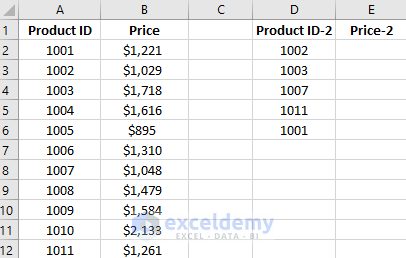Here we will use a formula by which we will get the corresponding price value of different product IDs. Write the formula =VLOOKUP(D2,\$A\$2:\$B\$12,2,FALSE) in cell E2.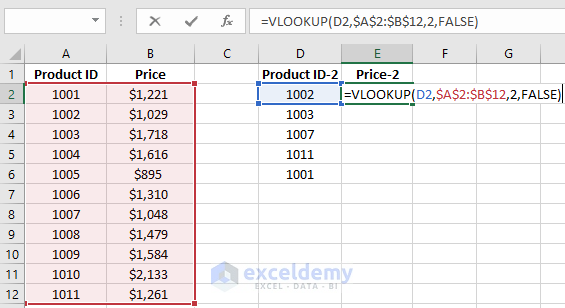After pressing Enter you will get the below result.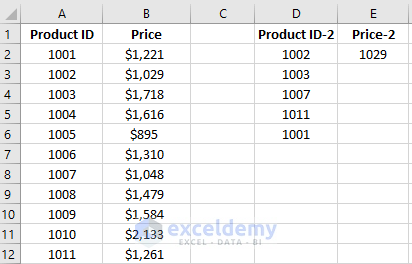Now, drag down the formulated cell E2 below from E2 to E6 to find out the result for the entire column.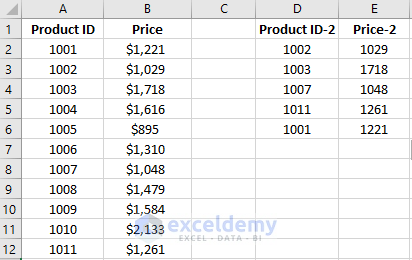## Matching Two Columns with Same Values to Return a Third Column (MATCH & INDEX)

The previous problem can be solved with a different formula. In this formula, we will be using the INDEX and MATCH function together. IT will give us the same output like the previous method.

To perform this write down the formula =INDEX(\$B\$2:\$B\$12, MATCH(D2,\$A\$2:\$A\$12)) in cell E2.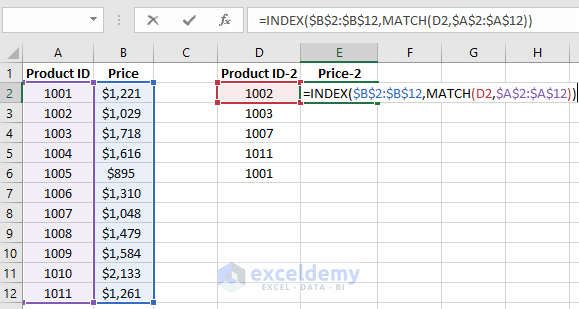After pressing enter you will get to see the below result.Now like before drag down this formulated cell in downwards to insert the formula for the whole column.## Using INDEX and MATCH Function to Match Two Columns in Excel and Return a Third

Let`s work on a different example where we will use two values from two different columns where the values exist in the same row. The return value will be from the third column and it will also be in the same row of the 2 values that will be given. Here we will be using a formula which contains the INDEX and MATCH Function. The below image shows the information of the example that we will be working on.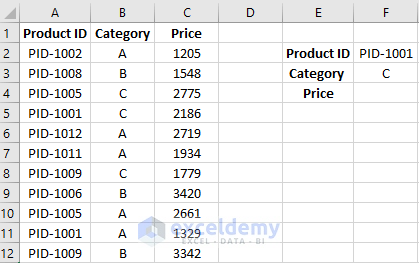Write down the formula, =INDEX(C2:C12,MATCH(F2,IF(B2:B12=F3,A2:A12),0)) in cell F4.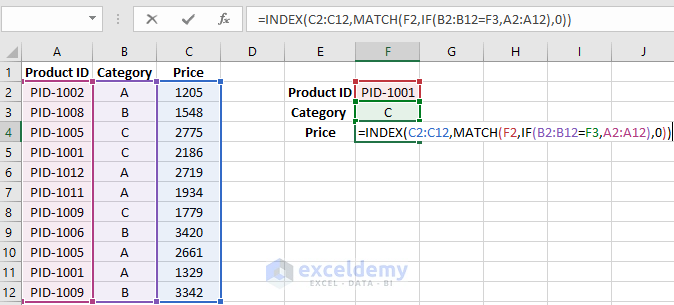After writing the formula press Ctrl + Shift +Enter to use it as an array formula. You will see a pair of 2nd brackets appear in the formula which contains the formula inside it. After doing this you will get to see the below result.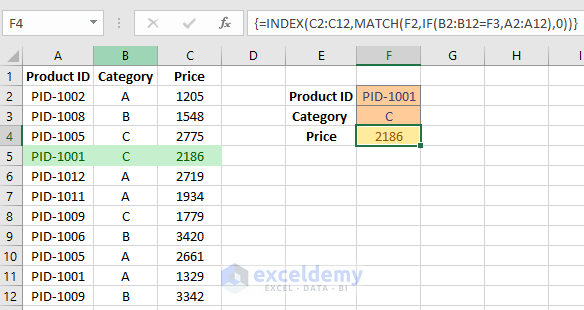Here the formula compares two values PID-1001 from column Product ID and C from column Category. From this, the resultant value is 2186 which is in the same row of these two values. By changing the cell values of cell F2 and F3 we will get different results in cell F4.

## Conclusion

Comparison between columns to find matches and resulting a value from a different column is a common practice in Excel. By knowing the solutions to this kind of problems makes your work easier in many cases. Hope you will like this article. Stay tuned for more useful articles.

## Related Articles

Hello!

Welcome to my Profile. Here I will be posting articles related to Microsoft Excel. I have completed my BSc in Electrical and Electronic Engineering from American International University-Bangladesh. I am a diligent, goal-oriented engineer with an immense thirst for knowledge and attitude to grow continuously. Continuous improvement and life-long learning is my motto.

1. ReplyEmil July 17, 2018 at 1:35 PM

the same can be done with SUMPRODUCT: SUMPRODUCT((A2:A12=F2)*(B2:B12=F3)*C2:C12)

• ReplyNishant December 19, 2019 at 10:22 PM

Better formula as you can compare more than just two cell, the only downside is that it will return with “0” and not #N/A which cause an issue if you are comparing data from two different time.

• ReplyJuly 17, 2018 at 2:38 PM

Thanks, Emil!

2. ReplyFerreira March 5, 2019 at 6:01 PM

Freat, I loved it.

3. ReplyMahendra Trivedi August 13, 2019 at 5:43 PM

How To Compare Two Columns with the single-cell And Return in multiple Values From The second Column In the single cell of Excel?